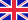•# State Constrained Optimal Control Problems

Autor: Svetlana Cherednichenko

In recent years the interest in using mathematical models for describing technological processes has been growing in different branches of economy. Often, these models involve partial differential equations, where technical restrictions are expressed as... Viac o knihe

Na objednávku, dodanie 2-4 týždne

42.84 €

bežná cena: 50.40 €

## O knihe

In recent years the interest in using mathematical models for describing technological processes has been growing in different branches of economy. Often, these models involve partial differential equations, where technical restrictions are expressed as inequality constraints. This work deals with constrained optimal control problems governed by partial differential equations. Solutions of such problems are usually approximated by sophisticated numerical methods, which require a discretization of the problem. In order to solve the discrete optimal control problem accurately, solutions should be validated by error estimates. Thus, efficient error estimates are crucial issues, since the reliability of numerical results can be expressed by corresponding convergence rates. Although theoretical and numerical foundations for control constrained problems are well studied, dealing with pure state constraints delivers certain difficulties on the analytical and computational sides. The work is addressed to mathematicians and engineers, working with constrained optimal control problems, in order to validate their numerical results and to find reasonable discretization.

• Vydavateľstvo: VDM Verlag
• Rok vydania: 2009
• Formát: Paperback
• Rozmer: 220 x 150 mm
• Jazyk: Anglický jazyk
• ISBN: 9783639147179

Generuje redakčný systém BUXUS CMS spoločnosti ui42.